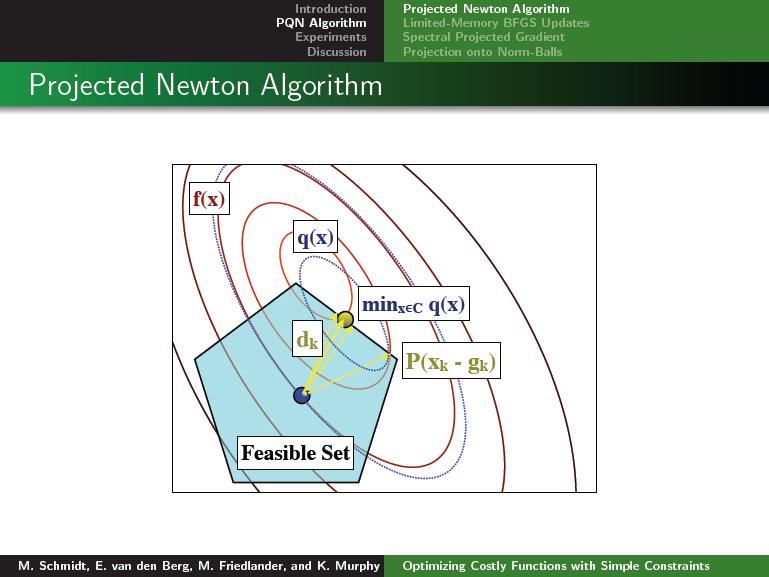# PQN: Optimizing Costly Functions with Simple Constraints

By Mark Schmidt, Ewout van den Berg, Michael Friedlander, Kevin Murphy (2009)
Last updated 3 July 2010.

## Summary

PQN implements a limited-memory projected quasi-Newton algorithm for constrained optimization. It uses L-BFGS updates to build a diagonal plus low-rank quadratic approximation to the function, uses the spectral projected gradient (SPG) algorithm to minimize the quadratic approximation subject to the constraints present in the original problem, and uses a backtracking line search to generate new parameter vectors satisfying an Armijo-like sufficient decrease condition. The method is most effective when computing the projection onto the constraint set can be done much more efficiently than evaluating the function.

The PQN method is decribed in the following paper:

Clicking on the image below opens the slides of a talk giving an overview of the method and results:PQN assumes that the function is differentiable, and that the user provides a function that computes the objective value (and optionally its gradient) for a given parameter vector. PQN also assumes that the set of values satisfying the constraints define a convex set, and that the user provides a function that computes the projection onto this set (ie. for a given parameter vector, this returns returns the closest parameter vector that satisfies the constraints).

• To use the code, download and unzip PQN.zip. Then, in Matlab, type:
```>> cd PQN
>> runSmall
```
Typing the above commands will run a set of experiments that are similar to those in the paper, except they run very quickly because they are on small data sets (where the differences between methods are very small).

We have included mex files for several operating systems. To compile the mex files for other operating systems, run the included mexAll function.

The function PQN_examples runs a series of demos illustrating the types of problems that PQN can solve. The full list of demos is:

• Linear Regression on the Simplex
• Lasso regression
• Lasso with Complex Variables
• Group-Sparse Linear Regression with Categorical Features
• Group-Sparse Simultaneous Regression
• Group-Sparse Multinomial Logistic Regression
• L_1,inf Blockwise-Sparse Graphical Lasso
• L_1,2 Blockwise-Sparse Graphical Lasso
• Linear Regression with the Over-Lasso
• Kernelized dual form of support vector machines
• Smooth (Primal) Support Vector Machine with Multiple Kernel Learning
• Conditional Random Field Feature Selection
• Approximating node marginals in undirected graphical models with variational mean field
• Multi-State Markov Random Field Structure Learning
• Conditional Random Field Structure Learning with Pseudo-Likelihood

The code, some documentation, and examples of the results of these demos can be found here.

## How to use the code

This code addresses the problem of minimizing a differentiable function f(x) over (closed) convex set X:
```min_x f(x), subject to x is in X
```
The PQN method is implemented in the Matlab function minConf_PQN. It can be called using the interface:
```>> x = minConf_PQN(funObj,x,funProj,options)
```
The first input funObj is a function handle that returns the objective value and gradient for a given parameter vector. For example, if we want to minimize the linear least squares objective
```f(x) = ||Ax - b||^2
```
we could use:
```>> funObj = @(x)SquaredError(x,A,b);
```
The second input, x, is the initial guess at the optimal solution (this guess doesn't need to satisfy the constraints). For example, we might use:
```>> p = size(A,2);
>> x = zeros(p,1);
```
The third input, funProj, returns the projection onto the convex set for a given parameter vector (ie. the closest point in X to the input point x). That is, for a given x the function funProj returns the unique solution to:
```P(x) = argmin_y ||y - x||, subject to y is in X
```
For example, if the constraints enforce that the variables must be non-negative we could use:
```>> funProj = @(x)boundProject(x,zeros(p,1),inf(p,1));
```
The last (optional) argument, options, is a structure that specifies if we want to change any of the default options. For example, we can reduce the number of L-BFGS corrections to store and turn on using numerical differentiation (instead of requiring that funObj return the gradient vector) using:
```>> options.corrections = 10;
>> options.numDiff = 1;
```
The full list of options can be accessed by typing help minConf_PQN, and includes things like changing the verbosity level of the program, changing the optimality tolerances, changing the maximum number of function evaluations or projections, and changing the parameters of the line search or SPG sub-routine.

## Reproducing the Paper Results

You can reproduce all of the figures in the paper using the plotAll function.

To re-run the experiments in the paper, use the runAll function.

This requires that you have the data sets available in the correct format. These data sets are not included in the zip file, they are available from the following sources: CoNLL 200 Chunking Task, Yeast Genomic Expression Data, Protein Signaling Data. The authors can be contacted about converting to our data format.

The machine running these experiments had 4GB of RAM, you may need to decrease 'options.corrections' if your machine hass less.

## Related Codes:

minConf - Other methods for multivariate differentiable optimization with different types of simple constraints
Gsparse - Matlab functions implementing Spectral Projected Gradient methods for optimization with a Group L1-norm constraint.
minFunc - Matlab function for unconstrained optimization of continuous real-valued multivariate functions (Examples).
SPGL1 - A solver for large-scale sparse reconstruction problems (Lasso, Basis Pursuit, Basis Pursuit Denoising)
crfChain - Matlab function implementing learning/inference/decoding/sampling in chain-structured conditional random fields with categorical features.
UGMlearn - Matlab code for structure learning in discrete-state undirected graphical models (Markov Random Fields and Conditional Random Fields) using Group L1-regularization.

Mark Schmidt > Software > PQN